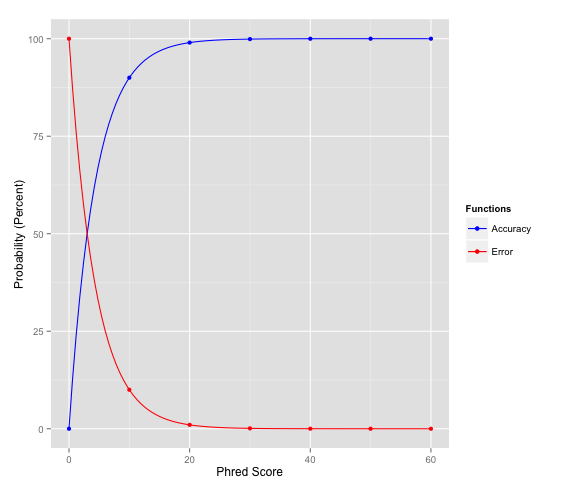# Phred-scaled Quality ScoresDictionary | Created 2014-06-05 | Last updated 2016-07-19

You may have noticed that a lot of the scores that are output by the GATK are in Phred scale. The Phred scale was originally used to represent base quality scores emitted by the Phred program in the early days of the Human Genome Project (see this Wikipedia article for more historical background). Now they are widely used to represent probabilities and confidence scores in other contexts of genome science.

### Phred scale in context

In the context of sequencing, Phred-scaled quality scores are used to represent how confident we are in the assignment of each base call by the sequencer.

In the context of variant calling, Phred-scaled quality scores can be used to represent many types of probabilities. The most commonly used in GATK is the QUAL score, or variant quality score. It is used in much the same way as the base quality score: the variant quality score is a Phred-scaled estimate of how confident we are that the variant caller correctly identified that a given genome position displays variation in at least one sample.

### Phred scale in practice

In today’s sequencing output, by convention, most useable Phred-scaled base quality scores range from 2 to 40, with some variations in the range depending on the origin of the sequence data (see the FASTQ format documentation for details). However, Phred-scaled quality scores in general can range anywhere from 0 to infinity. A higher score indicates a higher probability that a particular decision is correct, while conversely, a lower score indicates a higher probability that the decision is incorrect.

The Phred quality score (Q) is logarithmically related to the error probability (E).

$$Q = -10 \log E$$

So we can interpret this score as an estimate of error, where the error is e.g. the probability that the base is called incorrectly by the sequencer, but we can also interpret it as an estimate of accuracy, where the accuracy is e.g. the probability that the base was identified correctly by the sequencer. Depending on how we decide to express it, we can make the following calculations:

If we want the probability of error (E), we take:

$$E = 10 ^{-\left(\frac{Q}{10}\right)}$$

And conversely, if we want to express this as the estimate of accuracy (A), we simply take

$$\begin{eqnarray} A &=& 1 - E \nonumber \ &=& 1 - 10 ^{-\left(\frac{Q}{10}\right)} \nonumber \ \end{eqnarray}$$

Here is a table of how to interpret a range of Phred Quality Scores. It is largely adapted from the Wikipedia page for Phred Quality Score.

For many purposes, a Phred Score of 20 or above is acceptable, because this means that whatever it qualifies is 99% accurate, with a 1% chance of error.

Phred Quality Score Error Accuracy (1 - Error)
10 1/10 = 10% 90%
20 1/100 = 1% 99%
30 1/1000 = 0.1% 99.9%
40 1/10000 = 0.01% 99.99%
50 1/100000 = 0.001% 99.999%
60 1/1000000 = 0.0001% 99.9999%

And finally, here is a graphical representation of the Phred scores showing their relationship to accuracy and error probabilities.The red line shows the error, and the blue line shows the accuracy. Of course, as error decreases, accuracy increases symmetrically.

Note: You can see that below Q20 (which is how we usually refer to a Phred score of 20), the curve is really steep, meaning that as the Phred score decreases, you lose confidence very rapidly. In contrast, above Q20, both of the graphs level out. This is why Q20 is a good cutoff score for many basic purposes.